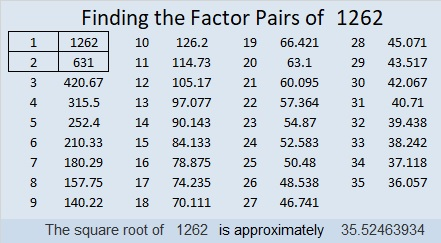# 1262 Jack-o-lantern Mystery

I have a week’s worth of Halloween themed mystery level puzzles starting with this jack-o-lantern. Mystery level doesn’t mean it’s difficult, only that I’m not letting you know if it’s tricky or not. You may find this puzzle is a real treat, so give it a try!Print the puzzles or type the solution in this excel file: 10-factors-1259-1270

Here are a few facts about the number 1262:

• 1262 is a composite number.
• Prime factorization: 1262 = 2 × 631
• The exponents in the prime factorization are 1 and 1. Adding one to each and multiplying we get (1 + 1)(1 + 1) = 2 × 2 = 4. Therefore 1262 has exactly 4 factors.
• Factors of 1262: 1, 2, 631, 1262
• Factor pairs: 1262 = 1 × 1262 or 2 × 631
• 1262 has no square factors that allow its square root to be simplified. √1262 ≈ 35.524641262 is the sum of the twenty-six prime numbers from 3 to 103.

This site uses Akismet to reduce spam. Learn how your comment data is processed.